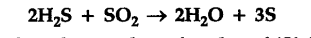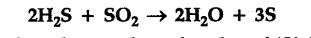# 0.5 mole each of \$H_{2}\$S and S\$O_{2}\$ mixed together in a reaction flask, react according to equation :

0.5 mole each of \$H_{2}\$S and S\$O_{2}\$ mixed together in a reaction flask, react according to equation :Calculate the number of moles of ‘S’ formed.Now, 2 moles of \${{H}{2}}S combine with 1 mole of S{{O}{2}} to give 3 moles of S. 1 mole of {{H}{2}}S combines with 0.5 mole of S{{O}{2}} to give 3/2 moles of S. Therefore, 0.5 moles of {{H}{2}}S combines with 0.25 mole of S{{O}{2}}\$ to give 3/2 x 0.5
= 0.75 moles of S.